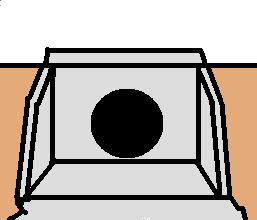* Log In to use the Calculate function * Become a Member!

###### Culvert Design:Culvert Design
Culvert Selection - Box
• Calculate the initial trial culvert size for the design of a box culvert.

Culvert Selection - Round
• Calculate the initial trial culvert size for the design of a round shaped culvert.
Culvert Selection - Other
• Calculate the initial trial culvert size for any shape culvert, including culvert shapes other than box and round.
HW - Submerged Inlet - IC
• Calculate the headwater depth (HW) for a culvert under inlet control (IC) with a submerged inlet.
HW - Unsubmerged Inlet - IC
• Calculate the headwater depth (HW) for a culvert under inlet control (IC) with an unsubmerged inlet.
Round Culvert - Head - OC
• Calculate the head (H) for a round culvert under outlet control (OC).
Arch Pipe - Head - OC
• Calculate the head (H) for an arch pipe culvert under outlet control (OC).
Square Box - Head - OC
• Calculate the head (H) for square box culvert under outlet control (OC).
Rectangle Box - Head - OC
• Calculate the head (H) for rectangle box culvert under outlet control (OC).
Any Shape - Head - OC
• Calculate the head (H) for any culvert with any cross section shape under outlet control (OC).
Tailwater Depth (ho)
• Calculate the tailwater depth below the top of outlet barrel for a culvert.
HW Depth - Outlet Control
• Calculate the headwater depth (HW) of a culvert under outlet control (OC).  Requires that the head (H) be calculated first by one of the above calculations.
Culvert Outlet Velocity
• Calculate the outlet velocity of a culvert.
Culvert Design Problem 1
• Size a concrete culvert pipe crossing under a county road for the following design conditions: Q = 35 cfs; maximum headwater elevation is 104.6; upstream flowline elevation is 100.1; downstream flowline elevation is 99.7; culvert length is 58 ft and the culvert will project from fill with no headwalls.

 Start Using The Calculations >>> Become A Member Now!